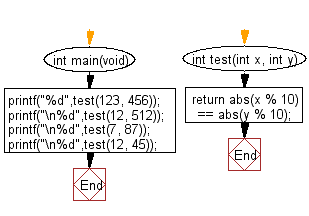﻿ C Program: Non-negative integers with same last digit# C Exercises: Check if two given non-negative integers have the same last digit

## C-programming basic algorithm: Exercise-13 with Solution

Write a C program to check if two given non-negative integers have the same last digit.

C Code:

``````#include <stdio.h>
#include <stdlib.h>

int main(void){
printf("%d",test(123, 456));
printf("\n%d",test(12, 512));
printf("\n%d",test(7, 87));
printf("\n%d",test(12, 45));

}
int test(int x, int y)
{
return abs(x % 10) == abs(y % 10);
}
``````

Sample Output:

```0
1
1
0
```

Pictorial Presentation:Flowchart:C Programming Code Editor:

What is the difficulty level of this exercise?

Test your Programming skills with w3resource's quiz.

﻿

## C Programming: Tips of the Day

Why doesn't a+++++b work?

printf("%d",a+++++b); is interpreted as (a++)++ + b according to the Maximal Munch Rule!.

++ (postfix) doesn't evaluate to an lvalue but it requires its operand to be an lvalue.

! 6.4/4 says the next preprocessing token is the longest sequence of characters that could constitute a preprocessing token"

Ref : https://bit.ly/3fdldUT